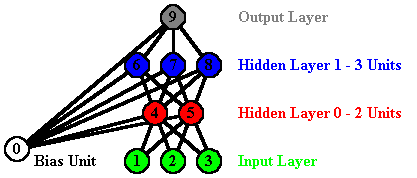### Introduction

In this lab you will be implementing the backpropagation algorithm (see the text book and class notes for more details on this algorithm). Your team assignments can be found here.

### MLPs and Backpropagation

In this assignment, you will be implementing a simple form of backpropagation network which we will call a multi-layer perceptron (or MLP for short). Your program will need to be able to construct and learn a neural network using backpropagation for different situations. To construct the network you will set various parameters, some of which will be contained in the code and a few that you will need to add yourself. For this code we will assume that your networks are limited to feed-forward networks with no cycles in the weights. Furthermore, you will assume that every hidden and output unit will be connected to a bias unit. For the remaining connectivity of the network you will use the MLP model. In this model, a network is viewed as containing several layers. Each network will have one input layer (corresponding to the input features) and one output layer (corresponding to the output features). There will also be some number of hidden layers (possibly 0) each with a number of units. For example, consider the following network:This network has an input layer with three units, an output layer with one unit and two hidden layers (the first with two units and the second with three). In an MLP, all of the units at one layer are always connected to all of the units in the previous layer (so, in the network shown all of the units in the first hidden layer are connected to the input units, all the units in the second hidden layer are connected to the first hidden layer and all the units in the output layer are connected to the second hidden layer). If there are no hidden layers, the output layer is connected directly to the input layer.

You will need to construct the user specified network (based on the options discussed below), train the network using those parameters and then be able to use that network in classification. You will also need to be able to read and write network files. The structure of the network will be given to you by the user in terms of the dataset (which will define the input and output layers) and in the options used to tell you about the hidden layer. The target output vectors can be determined using the routines get_output_vector_size and get_output_vector. You will need to write routines to do similar things to construct an input vector from an example.

### The Provided Code

The code you will be completing is in the archive smlp.tar.Z To use this code, download the archive to your account and then unpack it as follows:

```  uncompress smlp.tar.Z
tar xvf smlp.tar.Z
```

This will create the directory student_mlp and a set of files in that directory. The directory includes a makefile that will compile the provided files and produce three programs: train, classify and nfold. Unlike with previous assignments, I can not provide you with simple scripts to run these programs. To run the train program, you will need to provide at least the following arguments:

```  train -dataset c4.5 <NAMESFILE> <DATAFILE> -save model <MODELFILE> -learn mlp
```

You may also want to add "-report train predictions". Note that mlp has a number of options (discussed below). To run the classify program, you will need to provide at least the following arguments:

```  classify -dataset c4.5 <NAMESFILE> <DATAFILE> -use model <MODELFILE> -learn mlp
```

You may also want to add "-report predictions". To run the nfold program, you will need to provide at least the following arguments:

```  nfold -dataset c4.5 <NAMESFILE> <DATAFILE> -learn mlp
```

You may also want to add "-report test predictions".

The options currently available to you in the code are as follows (you can get this information also by simply typing train, classify or nfold with no arguments):